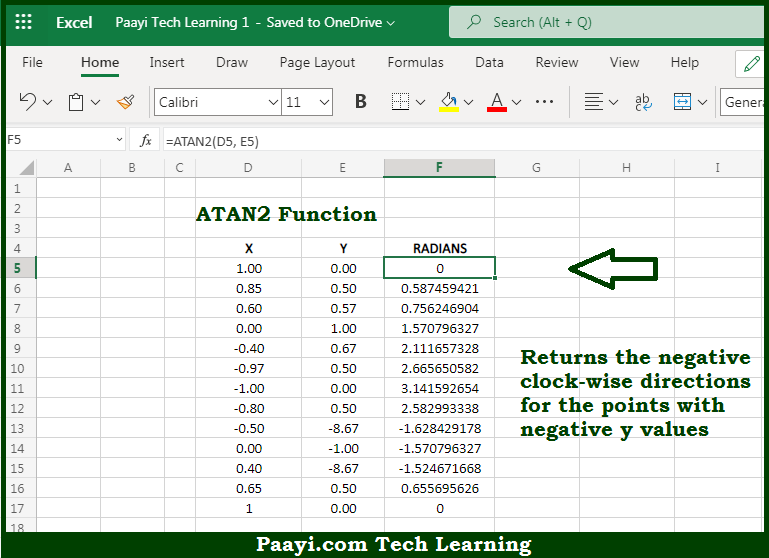# Learn How to Use Microsoft Excel ATAN2 Function

Written by | 0 Comments | 622 Views

In this article, you will learn how to use the Microsoft Excel ATAN2 function and its prime function in Microsoft Excel. You will also get to know the Microsoft Excel ATAN2 function return value and syntax with the help of some examples.

Microsoft Excel ATAN2 Function

The main purpose of the Microsoft Excel ATAN2 function is to get arctangent from x- and y- coordinates. That implies, with the help of the ATAN2 function you can able to return the arctangent from x- and y- coordinates. In geometry, ATAN2 returns the radian angle corresponding to the input point. So, with the help of the ATAN2 function, you can able to get the arctangent from x- and y- coordinates.

Return Value of ATAN2 Function

The return value will be the angle in radians of the point.

Syntax of ATAN2 Function

=ATAN2(x-num, y-num)

Where the arguments:

• x-num: This is the x coordinate of the input point.
• y-num: This is the y coordinate of the input point.

## How to Use Microsoft Excel ATAN2 Function?So we know that Microsoft Excel ATAN2 function you can able to get arctangent from x- and y- coordinates. That implies, with the help of the ATAN2 function you can able to return the arctangent from x- and y- coordinates. In geometry, ATAN2 returns the radian angle corresponding to the input point. So, with the help of the ATAN2 function, you can able to get the arctangent from x- and y- coordinates.